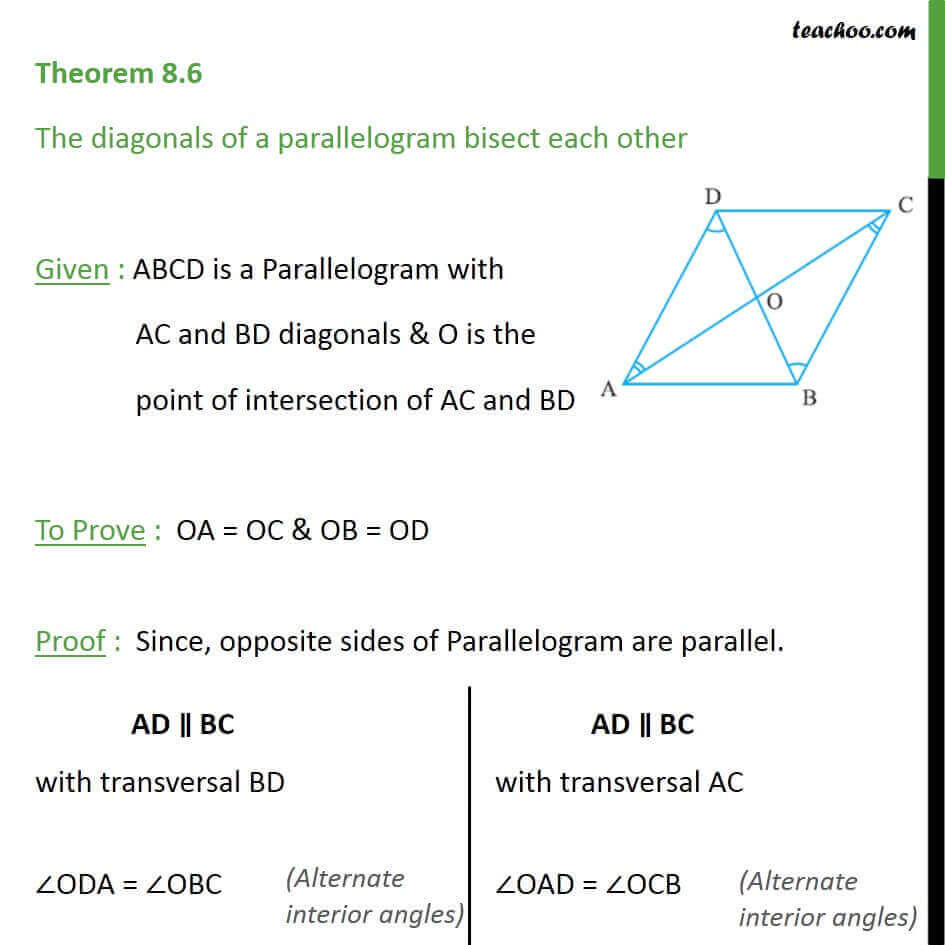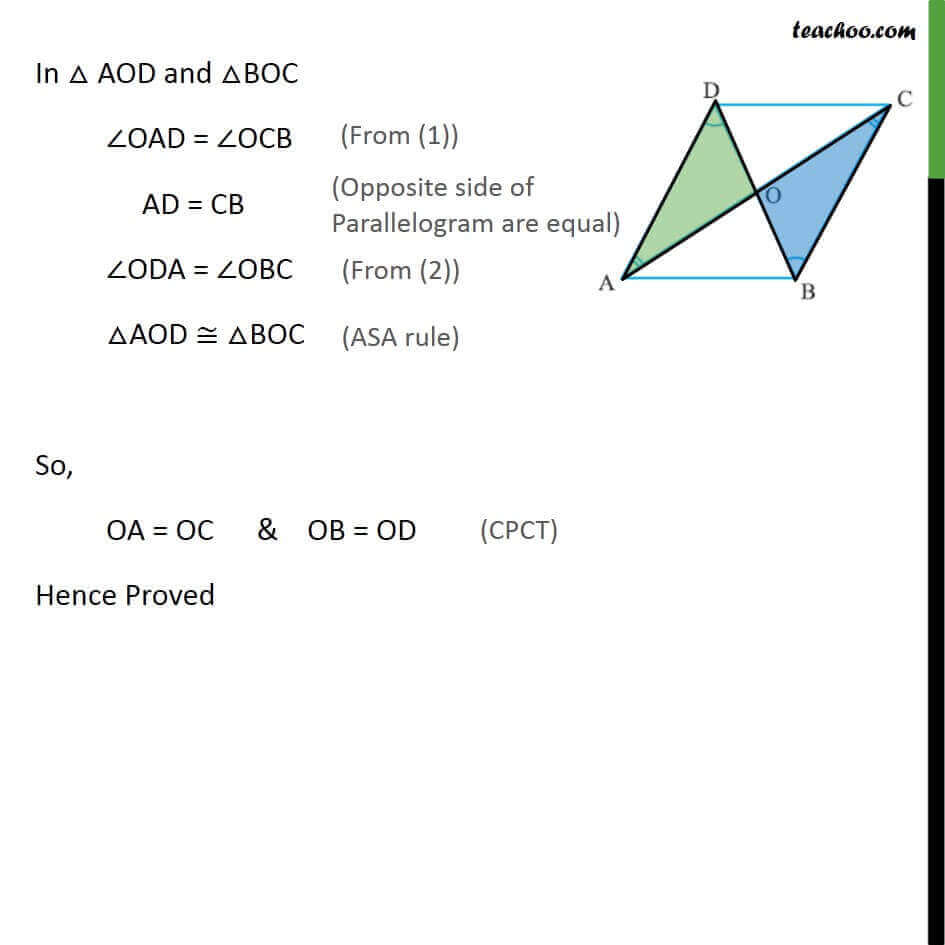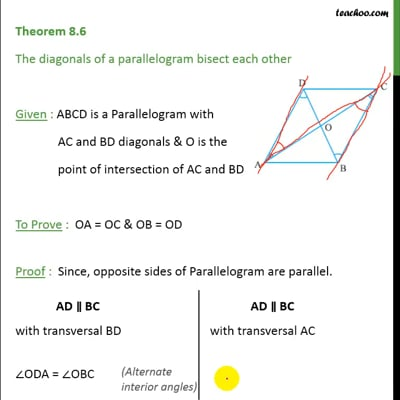Theorems

Serial order wiseThis video is only available for Teachoo black users

Introducing your new favourite teacher - Teachoo Black, at only ₹83 per month

### Transcript

Theorem 8.6 The diagonals of a parallelogram bisect each other Given : ABCD is a Parallelogram with AC and BD diagonals & O is the point of intersection of AC and BD To Prove : OA = OC & OB = OD Proof : Since, opposite sides of Parallelogram are parallel. In AOD and BOC OAD = OCB AD = CB ODA = OBC AOD BOC So, OA = OC & OB = OD Hence Proved